• PyTorch基础入门一：PyTorch基本数据类型1)Tensor(张量)Pytorch里面处理的最基本的操作对象就是Tensor(张量)，它表示的其实就是一个多维矩阵，并有矩阵相关的运算操作。在使用上和numpy是对应的，它和numpy唯一的...

PyTorch基础入门一：PyTorch基本数据类型

1)Tensor(张量)

Pytorch里面处理的最基本的操作对象就是Tensor(张量)，它表示的其实就是一个多维矩阵，并有矩阵相关的运算操作。在使用上和numpy是对应的，它和numpy唯一的不同就是，pytorch可以在GPU上运行，而numpy不可以。所以，我们也可以使用Tensor来代替numpy的使用。当然，二者也可以相互转换。

Tensor的基本数据类型有五种：

32位浮点型：torch.FloatTensor。pyorch.Tensor()默认的就是这种类型。

64位整型：torch.LongTensor。

32位整型：torch.IntTensor。

16位整型：torch.ShortTensor。

64位浮点型：torch.DoubleTensor。

那么如何定义Tensor张量呢？其实定义的方式和numpy一样，直接传入相应的矩阵即可即可。下面就定义了一个三行两列的矩阵：

import torch

# 导包

a = torch.Tensor([[1, 2], [3, 4], [5, 6]])

print(a)

不过在项目之中，更多的做法是以特殊值或者随机值初始化一个矩阵，就像下面这样：

import torch

# 定义一个3行2列的全为0的矩阵

b = torch.zeros((3, 2))

# 定义一个3行2列的随机值矩阵

c = torch.randn((3, 2))

# 定义一个3行2列全为1的矩阵

d = torch.ones((3, 2))

print(b)

print(c)

print(d)

Tensor和numpy.ndarray之间还可以相互转换，其方式如下：

Numpy转化为Tensor：torch.from_numpy(numpy矩阵)

Tensor转化为numpy：Tensor矩阵.numpy()

范例如下：

import torch

import numpy as np

# 定义一个3行2列的全为0的矩阵

b = torch.randn((3, 2))

# tensor转化为numpy

numpy_b = b.numpy()

print(numpy_b)

# numpy转化为tensor

numpy_e = np.array([[1, 2], [3, 4], [5, 6]])

torch_e = torch.from_numpy(numpy_e)

print(numpy_e)

print(torch_e)

之前说过，numpy与Tensor最大的区别就是在对GPU的支持上。Tensor只需要调用cuda()函数就可以将其转化为能在GPU上运行的类型。

我们可以通过torch.cuda.is_available()函数来判断当前的环境是否支持GPU，如果支持，则返回True。所以，为保险起见，在项目代码中一般采取“先判断，后使用”的策略来保证代码的正常运行，其基本结构如下：

import torch

# 定义一个3行2列的全为0的矩阵

tmp = torch.randn((3, 2))

# 如果支持GPU，则定义为GPU类型

if torch.cuda.is_available():

inputs = tmp.cuda()

# 否则，定义为一般的Tensor类型

else:

inputs = tmp

2)Variable(变量)

Pytorch里面的Variable类型数据功能更加强大，相当于是在Tensor外层套了一个壳子，这个壳子赋予了前向传播，反向传播，自动求导等功能，在计算图的构建中起的很重要的作用。Variable的结构图如下：import torch

# 定义三个Variable变量

x = Variable(torch.Tensor([1, 2, 3]), requires_grad=True)

w = Variable(torch.Tensor([2, 3, 4]), requires_grad=True)

b = Variable(torch.Tensor([3, 4, 5]), requires_grad=True)

# 构建计算图，公式为：y = w * x^2 + b

y = w * x * x + b

# 自动求导，计算梯度

y.backward(torch.Tensor([1, 1, 1]))

以上就是本文的全部内容，希望对大家的学习有所帮助，也希望大家多多支持我们。

时间： 2019-05-22

展开全文• 数据类型查看版本信息Tensor维度变换求导剥离无梯度变量Parameter 编程语言和自然语言一样，不理解的词越多，对全文的理解就越差。掌握必要的基础知识，让后期看代码更加流畅。 机器学习需要掌握数据处理工具Pandas...

数据类型

编程语言和自然语言一样，不理解的词越多，对全文的理解就越差。掌握必要的基础知识，让后期看代码更加流畅。

机器学习需要掌握数据处理工具Pandas、Numpy，同理，深度学习也需要掌握相应的工具，在Pytorch中数据存储在Tensor之中，本篇将介绍它们的基本用法以及与之相关的常用函数。

查看版本信息

包含头文件

import torch

1．查看torch版本

print(torch.__version__)

2．查看CUDA版本

print(torch.version.cuda)

GPU相关操作

1查看当前是否支持GPU

device = torch.device('cuda' if torch.cuda.is_available() else 'cpu')

2GPU相关的数据转换

a = torch.Tensor()
b = a.to('cuda') # 转成在 GPU 上运行的类型
b = a.cuda() # 同上
c = b.to('cpu') # 转成在 CPU 上运行的类型
c = b.cpu() # 同上

3 查看GPU状态

print(torch.cuda.device_count()) # 查看可用 GPU 个数
print(torch.cuda.current_device()) # 查看当前使用的 GPU ID
print(torch.cuda.get_device_capability(0)) # 查看 ID 为 0 的 CUDA 容量
print(torch.cuda.get_device_name(0)) # 查看设备名

4清空GPU缓存

print(torch.cuda.empty_cache()) # 清空缓存

Tensor

Tensor用于表示矩阵（多维数据），类似Numpy的ndarray，它可以使用GPU加速。从Pytorch 0.4.0之后，Variable与Tensor合并（Variable仍存在，但很少使用）。

1．生成Tensor

用Tensor方法将其它格式数据转换成张量，转换内容一般是list或数组格式：

a = torch.Tensor([[1,2],[3,4],[5,6]]) # 生成 3,2 维Tensor
b = torch.zeros(3,5) # 生成内容为全0的 3，5 维Tensor
c = torch.rand(3,5) # 生成内容为 0-1 随机数的 3,5 维Tensor
d = c.clone() # 将c中内容复制到 d, 新旧内容指向不同的地址空间

2．修改Tensor

用赋值的方法即可修改张量，比如将上面生成张量中位置1,1的元素赋值成50。

a[1,1]=50

3.查看Tensor状态

查看Tensor数据类型，大小及元素个数

1.  a = torch.Tensor()
2.  print(a.type()) # torch.FloatTensor，默认类型为FloatTesor
3.  print(a.size()) # torch.Size()
4.  print(a.shape)  # torch.Size()
5.  print(a.numel()) # 1，查看元素个数
6.  print(a.dim()) # 1, 查看维度

4．类型转换

Tensor与其它数据类型相互转换

1.  a = torch.Tensor() # tensor([5.])
2.  b = a.numpy()  # 转换成numpy.array类型 [5.]
3.  c = a.item() # 转换成单个值 5.0
4.  d = torch.from_numpy(b)  # 转换成Tensor tensor([5.])
5.  e = d.int() # 转换成 IntTensor tensor(, dtype=torch.int32)
6.  f = d.tolist() # 转换成list [5.0]

维度变换

1．增加维度

在深度学习过程中，现有的数据格式和模型要求的格式往往不同，层与层之间的数据也需要转换后才能对接，维度转换是最常用的方法。

squeeze意为压缩，即去掉维度，unsqueeze则相反，为添加维度。

unsqueeze(input, dim, out=None)

用于增添第dim维度为1。具体用法见以下示例：

1.  a = torch.Tensor([1,2,3])
2.  print(a, a.shape)  # tensor([1., 2., 3.]) torch.Size()
3.  b = torch.unsqueeze(a, 1)
4.  print(b, b.shape)  # tensor([[1.],[2.],[3.]]) torch.Size([3, 1])
5.  c = torch.unsqueeze(a, 0)
6.  print(c, c.shape)  # tensor([[1., 2., 3.]]) torch.Size([1, 3])

2．减小维度

1.  squeeze(input, dim=None, out=None)

用于删除第dim个维度，如果当前不包括指定的维度，则不会删除。如果不指定dim，函数将删除值为1的维度。

本例中延用上例中的数据：

1.  print(torch.squeeze(c,0))  # tensor([1., 2., 3.])
2.  print(torch.squeeze(b,1))  # tensor([1., 2., 3.])
3.  print(torch.squeeze(b))  # tensor([1., 2., 3.])

3．转换维度

比squeeze和unsqueeze更简单的方法是直接把张量转换成指定维度，使用view函数实现，它类似于numpy的reshape，Tensor也提供reshape方法。

1.  view(*shape)

将张量转换成指定的形状，示例：

1.  x = torch.Tensor([1,2,3,4])
2.  print(x.view(2,2))  # tensor([[1., 2.], [3., 4.]])
3.  print(x.view(1,4))  # tensor([[1., 2., 3., 4.]])
4.  print(x.view(1,-1)) # 设为-1时自动计算该维度大小 tensor([[1., 2., 3., 4.]])
5.  print(x.view(4))  # tensor([1., 2., 3., 4.])

4．cat拼接

cat函数用于在指定维度上拼接多个张量。

1.  cat(tensors, dim=0, out=None)

将tensors中的多个张量按dim指定的维度拼接成一个张量，拼接后总维数不变。

1.  x = torch.Tensor([[1,2],[3,4]])
2.  y = torch.Tensor([[5,6],[7,8]])
3.  print(torch.cat((x,y),0))
4.  # tensor([[1., 2.],
5.  #        [3., 4.],
6.  #        [5., 6.],
7.  #        [7., 8.]])
8.  print(torch.cat((x,y),1))
9.  # tensor([[1., 2., 5., 6.],
10. #        [3., 4., 7., 8.]])

一般面对的数据最多三维，并以一两维居多，可将其理解为横向加或者纵向加。

5．stack拼接

与cat拼接不同的是，stack拼接后维度增加，其用法如下：

1.  stack(tensors, dim=0, out=None)

示例：

1.  x = torch.Tensor([1,2])
2.  y = torch.Tensor([3,4])
3.  print(torch.stack((x,y),dim=0))
4.  # tensor([[1., 2.],
5.  #         [3., 4.]])
6.  print(torch.stack((x,y),dim=1))
7.  # tensor([[1., 3.],
8.  #         [2., 4.]])

从输出内容可以看到，拼接后张量变成了两维，dim=0是最常用的方法，它直接把两个张量拼在一起，当dim=1时，拼接时转换了位置。

6．transpose两维度互换

1.  transpose(input, dim0, dim1)

互换dim0, dim1两个维度，具体用法如下：

1.  x = torch.Tensor([[1,2],[3,4]])
2.  print(torch.transpose(x,0,1))
3.  # tensor([[1., 3.],
4.  #        [2., 4.]])

7．perumute多维度互换

permute与transpose功能类似，但更加灵活，它可以指定多个维度互换。

1.  x = torch.Tensor([[1,2],[3,4]])
2.  print(torch.transpose(x,0,1))
3.  # tensor([[1., 3.],
4.  #        [2., 4.]])

用于将张量转换成dim指定的维度。

1.  x = torch.rand(2,3,4)
2.  print(x.shape, x.permute(2,1,0).shape)
3.  # torch.Size([2, 3, 4]) torch.Size([4, 3, 2])

本例先产生了一组3维的随机数，每个维度的元素个数分别是2,3,4，然后使用permute将其第2维转成第0维，第1维不变，第0维转成第2维，从打印信息可以看到各维元素个数的变化。permute常用于对转换图片格式。

8．维度扩展

增加Tensor中元素的个数，其内容与被扩展的内容一致。

1.  a = torch.Tensor()
2.  print(a.expand(1, 2)) # tensor([[5., 5.]])

求导

下例中使用了torch.tensor而非torch.Tensor，torch.Tensor是Pytorch中的类，确切地说是FloatTensor的别名；而torch.tensor()是函数，它更加灵活，使用方法如下：

它可以创建各种类型的Tensor数据。

4.  y = a * x + b
5.  y.backward()
9.  print(b.data) # 输出结果: tensor([3.])

剥离无梯度变量

detach方法用于从普通Tensor中剥离出无梯度的变量。下面延用上例求导后有梯度的数据b。

2.  print(b.detach()) # tensor([3.])
3.  print(b.detach_()) # tensor([3.])

Parameter

常在两种场景中使用Parameter，一种是fine-tune精调时冻结模型中某些层的参数；另一种是自己实现一些特殊的结构，如构建Attention时，用Parameter创建需要调节的参数。

1．创建Parameter数据

1.  p = torch.nn.Parameter(torch.randn(2))
2.  print(p) # tensor([1.2087, 1.2607], requires_grad=True)

2.查看模型的Parameter参数，大小及冻结情况

1.  model = torch.nn.Linear(1,1)
2.  for name, param in model.named_parameters():
4.  # 输出结果:
5.  # weight torch.Size([1, 1]) True
6.  # bias torch.Size() True

感谢原文-知乎谢彦
Pytorch常用函数之一_数据类型

展开全文• 目录 python基础数据类型 numpy多维数组 ...由于在机器学习领域，python中的基础数据类型一般要转换成numpy中的多维数组或者torch的tensor来计算，本来简要描述其中的一些要点。https://tangshusen.me/...

目录

python基础数据类型

numpy多维数组

torch中的Tensor

torch中tensor操作

算术操作，以加法为例

索引操作

改变形状

运算内存开销

Tensor与numpy互相转换

tensor 转 numpy

numpy转tensor

tensor可以放到GPU上

由于在机器学习领域，python中的基础数据类型一般要转换成numpy中的多维数组或者torch的tensor来计算，本来简要描述其中的一些要点。https://tangshusen.me/Dive-into-DL-PyTorch/#/chapter02_prerequisite/2.2_tensor

python基础数据类型

严格来讲，python中是没有数组这个数据结构的，数组一般要求其中的元素类型形同。python中用来实现数组功能有两种基本数据类型，即列表list和元素tuple，其区别是前者是可变的，后者是不可变的。

python中赋值运算符与C++中有所不同，python中的赋值本质上是产生一个原对象的引用。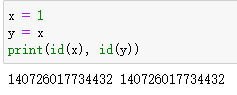id()可用于获取对象的内存地址，可以看到x和y的内存地址是相同的，即y得本质是x的一个引用。再看下边这个例子，如果y是x的引用，如果改变了y，那么x应该跟着变，但是这里y改变了 以后x并没有跟着变，并且x与y彻底分道扬镳，内存地址也不同了，变成了两个不同的对象。出现这种现象的原因是python六大基础类型中有可变对象与不可变对象之分，其中列表，集合，字典是可变类型，数字，字符串，元素是不可变类型，这里我们将一个不可变类型y改变数值时，会另外分配一块内存。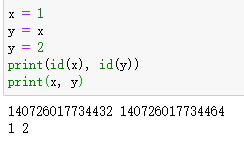如下图所示，改变一个不可变对象，会重新分配内存空间。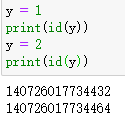再如先下边这个例子，尽管x是可变对象，不过y是x的一个元素的引用，所以y仍是数字类型的引用，因此改变y依然不影响x。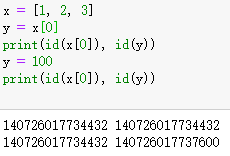但是当我们让y直接引用一个列表对象x时候，因为list是可变的，因此y的改变就会影响到x。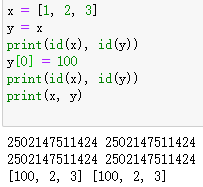一个比较有趣的点是python的数字类型Numbers只有int float bool complex这四种，而没有区分int和float的精度问题，据说可以认为是任意精度的。

numpy多维数组

numpy是python最常用的一个扩展库，主要用于矩阵运算，其最重要的一个数据结构是ndarray类型，即多维数组，要直接由python列表（或元组）创建一个多维数组只需要调用np.array(）函数就行。如下两个例子，由例子2也可以看出，从列表转换成numpy数组之后元素并没有共享空间。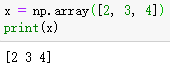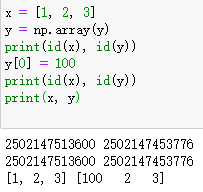此外还有一些生成指定形状多维数组的api：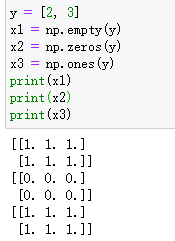其中np.empty()是创建指定形状数组的最快的方法，因为其不对数组内的元素进行初始化，即其中元素都是 随机值。

numpy中的数据类型比python内置类型多很多，也有数据精度的区分。具体可见https://www.runoob.com/numpy/numpy-dtype.html

此外numpy库中一个常用的库是numpy.random

https://blog.csdn.net/weixin_42029738/article/details/81977492

此外python标准库中也有random模块，与numpy中的random模块有一点区别。一般生成随机数，打乱一维数组等，可以用标准库中的random模块，而与随机数组相关的用numpy.random即可。

名叫random的模块有三个，python标准库中一个，numpy中一个，torch中也有一个。

torch中的Tensor

torch.Tensor是最重要的数据类型，更准确地说，Tensor是torch中默认张量对象FloatTensor的别名。

需要注意的是torch.Tensor()和torch.tensor()都可以用于生成张量对象，torch.tensor()则是一个函数，可以将python的内置数据类型list，tuple等，或者numpy数组转换成张量对象，且张量对象中数据类型由原对象数据类型决定，并不一定是单精度浮点数，另一个比较重要的特点是torch.tensor()生成张量是拷贝了原对象的数据，也就是说并不会跟原对象中的数据共享内存。

https://blog.csdn.net/weixin_42018112/article/details/91383574

张量相当于是numpy中的多维数组，只是其比多维数据还多了梯度等信息。

与numpy中接口类型，torch.empty()也可以生成未初始化的张量。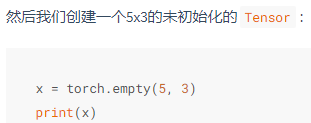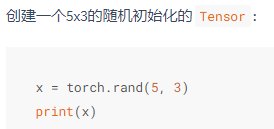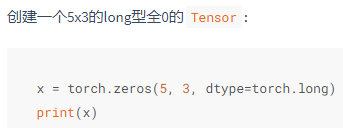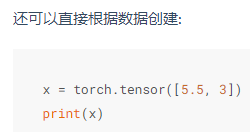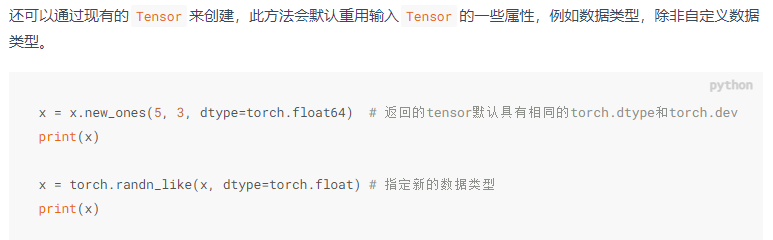可以用 x.size()方法或者x.shape成员来获取Tensor的形状（返回值的本质就是一个表示形状的元组）。

常用创建Tensor对象的api如下，跟numpy中创建多维数组也有很多相似之处啊。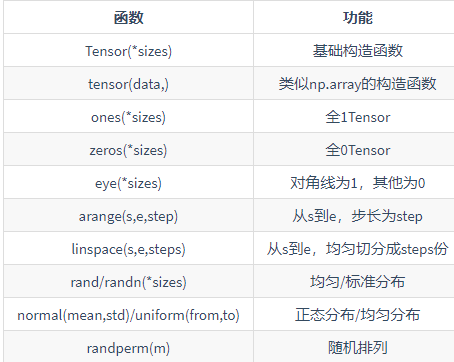torch中tensor操作

还可以进行原地加法运算。

索引操作

因为在动手学深度学习https://tangshusen.me/Dive-into-DL-PyTorch/#/chapter02_prerequisite/2.2_tensor上说对于tensor索引出来的结果与原数据共享内存，当经过下边两个例子验证，确实是这样子的，哪怕只索引了一个元素 x，他们的数据也是共享的，这点与python的标准数据类型是不同的。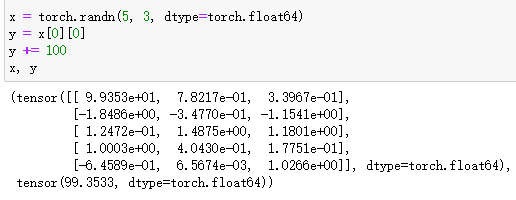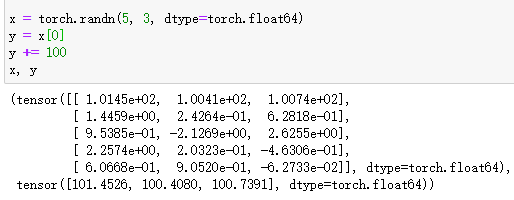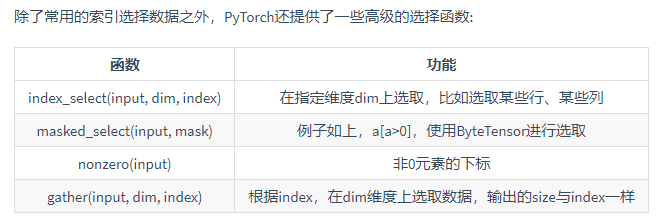改变形状

张量对象有一个view方法，可以用view来改变一个张量的形状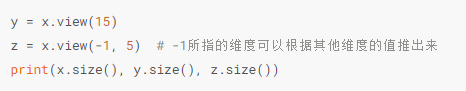但是用view改变得到的新形状的张量与原张量是共享数据的，即view只是改变了观察数据的角度，并没有实际上改变数据。

如果想要一个不共享数据的真正的副本，可以用reshape()函数来改变形状，但是此函数并不能保证返回的是拷贝，因此不推荐使用（reshape既有可能返回拷贝，也有可能跟view一样）。推荐使用clone创造一个副本然后再使用view。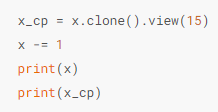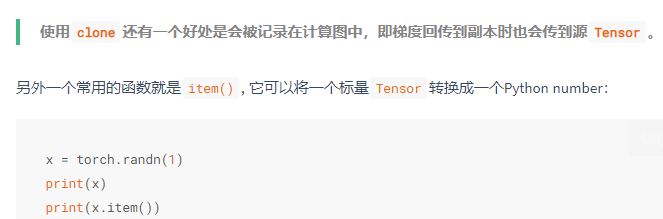运算内存开销

索引操作不开辟 新内存，而y = x + y这类操作是会开辟新内存的，可以通过索引操作来改写，使得y = x + y不开辟新内存，如下所示。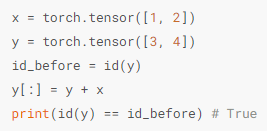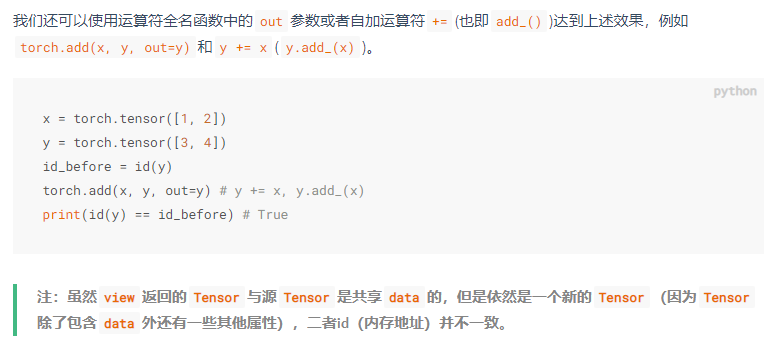Tensor与numpy互相转换

tensor 转 numpy

tensor对象有一个numpy()成员方法，直接a.numpy()即可，且这种方法产生的numpy数组与原张量的数据是共享内存的，即一个改变另一个也改变。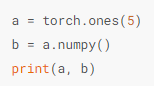numpy转tensor

torch中有一个from_numpy()函数，这样转换得到的tensor与原numpy数组也是共享内存的。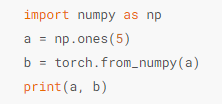还有一个方法是直接用torch.tensor()，但是这种方法会进行数据拷贝，开辟新内存。tensor可以放到GPU上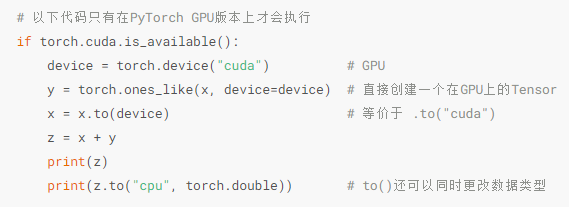展开全文python
• Pytorch 数据类型与转换

万次阅读 2019-05-20 12:43:23
torch定义了7种CPU tensor类型和八种GPU tensor类型： Data tyoe CPU tensor GPU tensor 32-bit floating point torch.FloatTensor torch.cuda.FloatTensor 64-bit floating point torch....

torch定义了7种CPU tensor类型和八种GPU tensor类型：

Data tyoeCPU tensorGPU tensor
32-bit floating pointtorch.FloatTensortorch.cuda.FloatTensor
64-bit floating pointtorch.DoubleTensortorch.cuda.DoubleTensor
16-bit floating pointN/Atorch.cuda.HalfTensor
8-bit integer (unsigned)torch.ByteTensortorch.cuda.ByteTensor
8-bit integer (signed)torch.CharTensortorch.cuda.CharTensor
16-bit integer (signed)torch.ShortTensortorch.cuda.ShortTensor
32-bit integer (signed)torch.IntTensortorch.cuda.IntTensor
64-bit integer (signed)torch.LongTensortorch.cuda.LongTensor

使用时，直接传入数字，就是按照形状初始化。

torch.FloatTensor(2,3)
torch.DoubleTensor(2,3)
torch.ByteTensor(2,3)
torch.CharTensor(2,3)
torch.ShortTensor(2,3)
torch.IntTensor(2,3)
torch.LongTensor(2,3)

也可以，传入一个[2,3]这样的序列，就会被当成数组处理。

与dtype的对应关系:

FloatTensor ->  'float32'

DoubleTensor -> 'float64'

ShortTensor -> 'int16'

IntTensor -> 'int32'

LongTensor -> 'int64'

可以自行用代码测试：

import torch

a= torch.FloatTensor(1)

print(a.dtype)
#'float32'

不同dtype之间原则上不能混合运算。

所以我经常遇到'float64' * 'float32' 报错。

RuntimeError: expected type torch.DoubleTensor but got torch.FloatTensor

你可以对一个tensor进行强行转换。

import torch

t= torch.Tensor(3.14159265)

t = t.float()    #'float32'
t = t.double()   #'float64'
t = t.int()      #'int32'

也可以对模型进行这个骚操作。

比如下面的代码，让lstm的输出从原来的'float32'变成'float64'，这就便于后面与其他'float64'的运算。

self.lstm = nn.LSTM(input_size=self.emb_dim,
hidden_size=n_lstm_units,
dropout=1-keep_prob).double()

请问，直接改变Tensor的dtype，会不会影响梯度的backward？

pytorch官方成员回答，不会。

@albanD:

Change of types are considered as any other operations by the autograd engine and so all the gradients will be computed properly. Note that there might be a loss of precision of going from double to float as you would expect.

展开全文•机器学习 深度学习 pytorch 基本数据类型pytorch 深度学习 机器学习
• torch.dtype是表示torch.Tensor的数据类型的对象。PyTorch有八种不同的数据类型： 使用方法： （1）定义一个tensor torch.tensor(data, dtype=None, device=None, requires_grad=False, pin_memory=False) → ...
• 在PyTorch中，传入网络计算的数据类型必须是 Variable 类型， Variable包装了一个Tensor，并且保存着梯度和创建这个Variable function的引用，换句话说，就是记录网络每层的梯度和网络图，可以实现梯度的反向传递。...
• 一、张量的数据类型、默认类型、类型转换。 二、张量的生成：torch.tensor()、torch.Tensor()、张量和NumPy数据互相转换、随机数生成张量、函数生成等。 三、张量操作：改变张量的形状、获取张量中的元素、拼接和...python 深度学习
• Torch建立数据迭代器进行网络模型训练的两种方法数据库的建立方法一方法二 数据库的建立 数据格式要求：.csv文件，第一列为标签，第二列之后为数据 建立数据库代码 import torch import pandas as pd import torch....pycharm
• 1.6.2.Pytorch的基础数据类型 1.6.2.1.Torch定义了的七种CPU tensor类型和八种GPU tensor类型 1.6.2.2.基础数据类型 1.6.2.3.Pytorch数据类型 1.6.2.4.判断数据类型 1.6.2.5.CPU或GPU张量之间的转换 1.6.2.6.数据...
• 深度学习其实就是一个最优化问题，找到最小的loss值，因为自变量过多，想要找到最小值非常困难。所以就出现了很多最优化...tensor还可以指定数据类型，以及数据存储的位置（可以存在显存里，硬件加速） torch.te...深度学习 神经网络 机器学习 人工智能 python
• 实际上，Variable是Torch里的一种数据类型，他与tensor没有什么很大的区别，但 是他们属于不同的数据类型，Variable对象有更多的属性。主要的区别如下： ①、Variable类型的变量具有自动求导的功能 ②、Variable类型...
• 这是一个适合PyTorch入门者看的博客。PyTorch的文档质量比较高，入门较为容易，这篇博客选取官方链接里面的例子，介绍如何用PyTorch训练一个ResNet模型用于图像...解释的思路是从数据导入开始到模型训练结束，基本上...
• PyTorch基础入门一：PyTorch基本数据类型

万次阅读 多人点赞 2018-07-28 11:14:05
PyTorch基础入门一：PyTorch基本数据类型 1）Tensor(张量) Pytorch里面处理的最基本的操作对象就是Tensor（张量），它表示的其实就是一个多维矩阵，并有矩阵相关的运算操作。在使用上和numpy是对应的，它和numpy...深度学习 人工智能 pytorch
• NumpyNumpy----》Tensor1.6 Tensor在GPU上的使用1.7 自动求梯度1.7.1 概念1.7.2 Tensor1.7.3 梯度中断梯度追踪 说明：本博客是对如何使用pytorch用于深度学习 学习过程的记录和总结。 学习教程为：《动手学深度学习...python 深度学习 自然语言处理 pytorch 神经网络
• PyTorch中的常用的tensor类型PyTorch中的常用的tensor类型包括：32位浮点型torch.FloatTensor，64位浮点型torch.DoubleTensor，16位整型torch.ShortTensor，32位整型torch.IntTensor，64位整型torch.LongTensor。...
• pytorch中有时需要复制一下张量（tensor），如果实在迭代过程中就会涉及到梯度的问题。 我了解的常用的tensor复制方式有两种 .detach（）和.clone（） 1、.detach（）方法，比如已有tensor a ，b=a.detach（），则...
• 参考https://zhuanlan.zhihu.com/p/32154263 通过tensorboard检测梯度值和参数值来查找原因。
• import d2lzh1981 as d2l torch.set_default_tensor_type(torch.FloatTensor) 获取和读取数据集 比赛数据分为训练数据集和测试数据集。两个数据集都包括每栋房子的特征，如街道类型、建造年份、房顶类型、地下室状况...
• 我们可以通过指定参数reuqires_grad=True来建立一个反向传播图，从而能够计算梯度。在pytorch中一般叫做dynamic computation graph(DCG)——即动态计算图。import torchimport numpy as np# 方式一x = torch.randn(2...
• 初学者的CNN搭建示例(torch,cifar10数据集)前言： 之前一直眼高手手低，哦是懒，也就是偶尔翻翻书，不怎么摸代码更不用说project了。 本硕都是电气，硕士快结束喜欢上AI这东西，半路出家就搞起了机器学习，原因呢很...torch7 cnn神经网络 cifar-10
• 正如numpy包中的array，Pandas包中的Series和Dataframe一样，为了方便计算，Pytorch也在Python数据结构的基础上封装了几个基本的数据结构。 一、张量（Tensor） 没错，就是TensorFlow名字里的Tensor，本来谷歌给...pytorch python 人工智能
• 在《动手学深度学习》中，将使用torch.Tensor作为存储和变换数据的主要工具   Tensor与Numpy相比，主要优势在于：与Numpy功能类似，并提供GPU计算和自动求梯度等更丰富的功能 1.1 初始化   1）torch.empty：创建...深度学习 机器学习 python
• 手写数字识别大致思路 线性模型 添加非线性部分 只有线性部分的计算是很难完成识别任务的，因此我们需要添加非...看看如何用梯度下降的方法解决 再使用argmax函数得到索引值。 import torch from torch import nn深度学习 神经网络 python pytorch torch
• 4.5 PyTorch批量梯度下降

千次阅读 2020-02-15 13:54:52
第二章：认识Tensor的类型、创建、存储、api等，打好Tensor的基础，是进行PyTorch深度学习实践的重中之重的基础。 第三章：学习PyTorch如何读入各种外部数据 第四章：利用PyTorch从头到尾创建、训...pytorch
• Pytorch中的Tensor常用的类型转换函数(inplace操作)：(1)数据类型转换在Tensor后加 .long(), .int(), .float(), .double()等即可，也可以用.to()函数进行转换，所有的Tensor类型可参考...----> GPU张量......